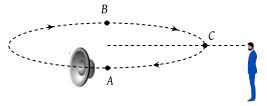If source and observer both are relatively at rest and if speed of sound is increased then frequency heard by observer will :

(1) Increases

(2) Decreases

(3) Can not be predicted

(4) Will not change

Concept Questions :-

Doppler effect
High Yielding Test Series + Question Bank - NEET 2020

Difficulty Level:

A source and an observer move away from each other with a velocity of 10 m/s with respect to ground. If the observer finds the frequency of sound coming from the source as 1950 Hz, then actual frequency of the source is (velocity of sound in air = 340 m/s)

(1) 1950 Hz

(2) 2068 Hz

(3) 2132 Hz

(4) 2486 Hz

Concept Questions :-

Doppler effect
High Yielding Test Series + Question Bank - NEET 2020

Difficulty Level:

A whistle revolves in a circle with an angular speed of 20 rad/sec using a string of length 50 cm. If the frequency of sound from the whistle is 385 Hz, then what is the minimum frequency heard by an observer, which is far away from the centre in the same plane ?

(1) 333 Hz

(2) 374 Hz

(3) 385 Hz

(4) 394 Hz

Concept Questions :-

Doppler effect
High Yielding Test Series + Question Bank - NEET 2020

Difficulty Level:

A Siren emitting sound of frequency 800 Hz is going away from a static listener with a speed of 30 m/s, frequency of the sound to be heard by the listener is (take velocity of sound as 330 m/s)

(1) 733.3 Hz

(2) 644.8 Hz

(3) 481.2 Hz

(4) 286.5 Hz

Concept Questions :-

Doppler effect
High Yielding Test Series + Question Bank - NEET 2020

Difficulty Level:

A car sounding a horn of frequency 1000 Hz passes an observer. The ratio of frequencies of the horn noted by the observer before and after the passing of the car is 11 : 9. If the speed of sound is v, the speed of the car is

(1) $\frac{1}{10}v$

(2) $\frac{1}{2}v$

(3) $\frac{1}{5}v$

(4) v

Concept Questions :-

Doppler effect
High Yielding Test Series + Question Bank - NEET 2020

Difficulty Level:

A small source of sound moves on a circle as shown in the figure and an observer is standing on O. Let n1, n2 and n3 be the frequencies heard when the source is at A, B, and C respectively. Then(1) n1 > n2 > n3

(2) n2 > n3 > n1

(3) n1 = n2 > n3

(4) n2 > n1 > n3

Concept Questions :-

Doppler effect
High Yielding Test Series + Question Bank - NEET 2020

Difficulty Level:

A source emits a sound of frequency of 400 Hz, but the listener hears it to be 390 Hz. Then

(1) The listener is moving towards the source

(2) The source is moving towards the listener

(3) The listener is moving away from the source

(4) The listener has a defective ear

Concept Questions :-

Doppler effect
High Yielding Test Series + Question Bank - NEET 2020

Difficulty Level:

A person carrying a whistle emitting continuously a note of 272 Hz is running towards a reflecting surface with a speed of 18 km/hour. The speed of sound in air is 345 ms–1. The number of beats heard by him is

(1) 4

(2) 6

(3) 8

(4) 3

Concept Questions :-

Beats
High Yielding Test Series + Question Bank - NEET 2020

Difficulty Level:

If the pressure amplitude in a sound wave is tripled, then the intensity of sound is increased by a factor of

(1) 9

(2) 3

(3) 6

(4) $\sqrt{3}$

Concept Questions :-

Energy of waves
High Yielding Test Series + Question Bank - NEET 2020

Difficulty Level:

If the amplitude of the sound is doubled and the frequency reduced to one-fourth, the intensity of sound at the same point will be :

(1) Increased by a factor of 2

(2) Decreased by a factor of 2

(3) Decreased by a factor of 4

(4) Unchanged

Concept Questions :-

Energy of waves